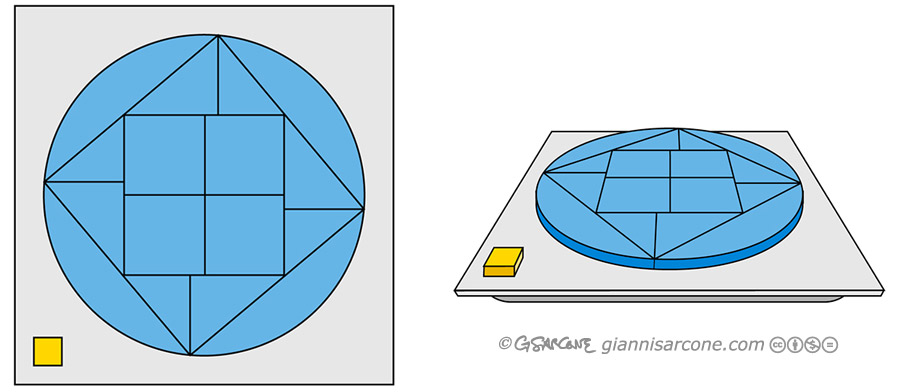### Rolling Polygons

Imagine the wheels of your bike are polygons. Then, to ride smoothly the road should be made of ‘catenaries‘ (yes, those bumpy things).It is the math professor Stan Wagon who first demonstrated this concept with a real square-wheel bike at Macalester College in St. Paul, Minnesota. Continue reading “Rolling Polygons”

### Linear to rotational motion

Intriguing linear motion perceived as circular motion! Watch as the black balls rotate in a circle, then focus on one ball at a time and you will notice that it follows a straight line. Also, watch at the moment when there are only four balls moving, it forms a rotating square between the four balls. This is just neat example of looking deeper into something so simple and discovering a hidden pattern.More

Pattern with Arabesque paths moving in a linear fashion induces rotational motion to a hexagonal device.

### “Magic” Factorials

There are many fun facts regarding the factorials. For instance:

• 0! = 1 by convention. As weird as it may sound, this is a fact that we must remember.
• The number of zeroes at the end of n! is roughly n/4.
• 70! is the smallest factorial larger than a googol.
• The sum of the reciprocals of all factorials is e.
• Factorials can be extended to fractions, negative numbers and complex numbers by the Gamma function.

It is possible to “peel” each layer off of a factorial and create a different factorial, as shown in the neat number pattern below.A prime pattern can be found when adding and subtracting factorials. Alternating adding and subtracting factorials, as shown in the picture, yields primes numbers until you get to 9! Continue reading ““Magic” Factorials”

### Haruki’s Theorem

Given 3 circles, each intersecting the other two in two points, the line segments connecting their points of intersection satisfy: ace/bdf = 1### Morley’s Trisector Theorem

In any triangle, the 3 points of intersection of the adjacent angle trisectors ALWAYS form an equilateral triangle (in blue), called the Morley triangle.### Surprising Limit

Amazingly, this sequence of fractions converges to 0.70710678118…, or to be precise, to √2/2. The sequence is related to the Prouhet-Thue-Morse sequence.### Prime Fractions

Did you know? You can write the number 1 as a sum of 48 different fractions, where every numerator is 1 and every denominator is a product of exactly two primes.This problem is related to the Egyptian fractions.

### Very Large Numbers In Real LifeContinue reading “Math-Magic Vanishing Space”